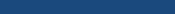Valdir Aguilera# E = m c2

Albert Einstein

It followed from the special theory of relativity that mass and energy are both but different manifestation of the same thing – a somewhat unfamiliar conception for the average mind. Furthermore, the equation E is equal to m c squared, in which energy is put equal to mass, multiplied by the square of the velocity of light, showed that very small amounts of mass may be converted into a very large amount of energy and vice versa. The mass and energy were in fact equivalent, according to the formula mentioned before. This was demonstrated by Cockcroft and Walton in 1932, experimentally.

Para ouvi-lo, clique AQUI.

Segue-se, da Teoria Especial da Relatividade, que massa e energia são ambas manifestações diferentes de uma mesma coisa – um conceito de certa forma não familiar para as mentes médias. Além disso, a equação E é igual a m c quadrado, onde a energia é expressa como massa multiplicada pelo quadrado da velocidade da luz, mostrou que quantidades de massa muito pequenas podem ser convertidas em quantidades de energia muito grandes e vice-versa. Massa e energia são, de fato, equivalentes, de acordo com a fórmula mencionada antes. Isso foi demonstrado experimentalmente por Cockcroft e Walton em 1932.

De "Einstein" para "Biblioteca"